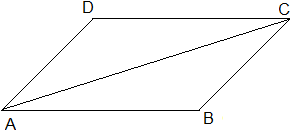# In the parallelogram ABCD the area of ΔABC is 10 sq.cm then the area of ABCD = ………………. sq.cm

17 views

closed

In the parallelogram ABCD the area of ΔABC is 10 sq.cm then the area of ABCD = ………………. sq.cmA) 15

B) 40

C) 20

D) 10

+1 vote
by (56.9k points)
selected by

Correct option is (C) 20In parallelogram ABCD, AC is a diagonal.

$\because$ area of $\triangle ABC$ = area of $\triangle ACD$

Also, area of $\triangle ABC$ + area of $\triangle ACD$ = area of rectangle ABCD.

$\therefore$ Area of rectangle ABCD $=2\times$ Area of $\triangle ABC$

$=2\times10\,cm^2$

$=20\,cm^2$

by (42.4k points)

Correct option is  C) 20